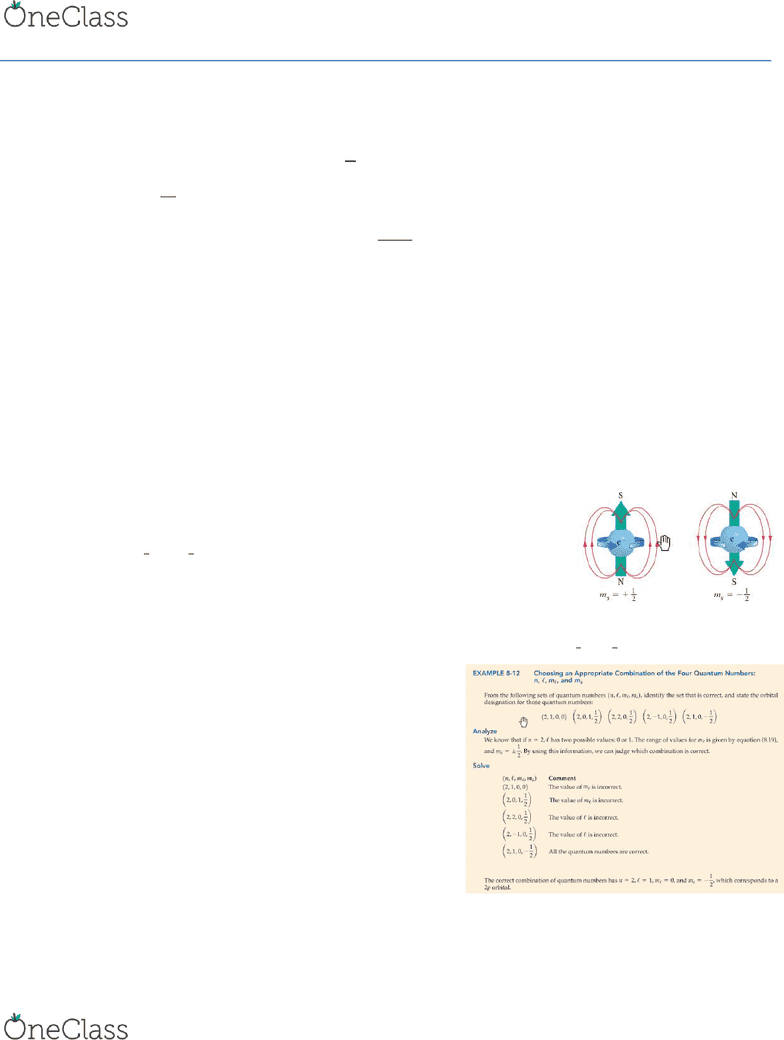Textbook Notes (280,000)
CA (170,000)
Queen's (4,000)
CHEM (40)
Chapter 8.7-8.12 and 9.1=9.7

# CHEM 112 Chapter Notes - Chapter 8.7-8.12 and 9.1=9.7: Unpaired Electron, Pauli Exclusion Principle, Electron Configuration

Department
Chemistry
Course Code
CHEM 112
Professor
Gang Wu
Chapter
8.7-8.12 and 9.1=9.7

This preview shows page 1. to view the full 4 pages of the document.Chemistry Week 3
8-7
Interpreting and representing the orbitals of the hydrogen atom
The square of the wave function relates to the probability density of three dimensional shapes
The angular part of the wave functions for an s-orbital
 is always the same regardless of the principal quantum number
o Thus all the orbitals of a given type (s,p,d,f) have the same angular behavior
The term is equal to 

o the quantity is equal to the Bohr Radius, the value of which can be related to other constants in the Schrödinger equation
 
o To obtain the wave function of a particular state, we must multiply the angular part by the radial part
o Determines how the probability density for a particular state changes with distance from the nucleus
o Each radial function decays exponentially with increasing radius of an orbital and sometimes cross the horizontal axis before settling at 0
The radial function decays as r increases, thus the larger the n value, the larger the orbital
The radial function crosses the horizontal axis n - - 1 times before hitting 0
For s orbitals the radial factor has a non-zero value at the nucleus but the p,d and f orbitals have a 0 value at the nucleus
The probability density for s orbitals is max at r=0
The probability density for p,d and f is 0 at r=0
Angular functions
8-8
Electron spin a fourth quantum number ()
Proposed based on some unexplained feature of the hydrogen spectrum that an electron spins on an axis
Two possibilities

Explanation of magnetic spin
o An electron, because of its spin, generates a magnetic field
o A pair of electrons with opposing spins has no magnetic field
o A silver atom in its lowest energy state has only one unpaired electron. The direction of the net magnetic field produced depends only on
the spin for the unpaired electrons
o In a bean of silver atoms, there is an equal chance that the unpaired electron will have a spin of

Electron structure of the H atom; representing the four quantum numbers
8-9
Multielectron atoms
A conceptual model for multielectron atoms
1. Each orbital in a multielectron atom describes how a single electron
behaves in a field of a nucleus under the average influence of all other
electrons
2. The total energy of an atom with N electrons has the general form of
   , where F= sum of all orbital energies and G=electron-
electron repulsions
As n increases ℓ increases
3. The order in which we assign electrons to specific orbitals is based on
minimizing Eatom
Penetration and shielding
o Electrons in orbitals closer to the nucleus shield other electrons from getting to the nucleus
o Screening electrons reduce the effectiveness of the nucleus in attracting the particular more-distant electrons
o They effectively reduce the nuclear charge felt by the more-distant electron
o S-orbitals are the best at screening because they have a high probability density at the nucleus
o The nuclear charge that an electron actually expenses is reduced by intervening electrons to a value of Zeff called the effective nuclear
charge
find more resources at oneclass.com
find more resources at oneclass.com